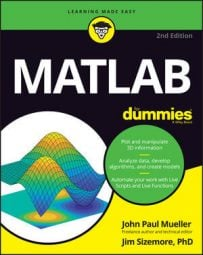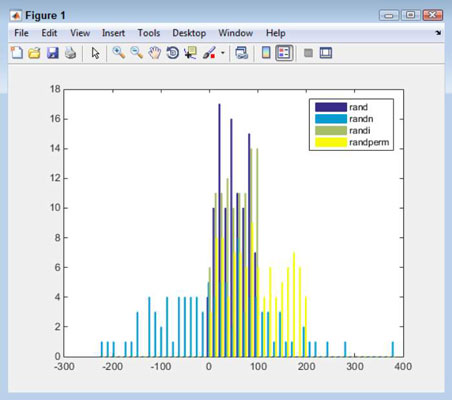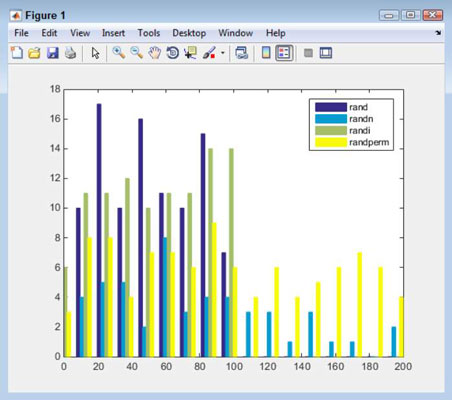##### MATLAB For DummiesMATLAB provides a number of commands that you can use to perform basic statistics tasks. When working with descriptive statistics, the math quantitatively describes the characteristics of a data collection, such as the largest and smallest values, the mean value of the items, and the average. This form of statistics is commonly used to summarize the data, thus making it easier to understand.

The following steps help you work through some of these tasks:

1. Type rng(‘shuffle', ‘twister'); and press Enter.

You use the rng() function to initialize the pseudo-random number generator to produce a sequence of pseudo-random numbers. Older versions of MATLAB use other initialization techniques, but you should rely on the rng() function for all new applications.

The first value, shuffle, tells MATLAB to use the current time as a seed value. A seed value determines the starting point for a numeric sequence so that the pattern doesn’t appear to repeat. If you want to exactly repeat the numeric sequence for testing purposes, you should provide a number in place of shuffle.

The second value, twister, is the number generator to use. MATLAB provides a number of these generators so that you can further randomize the numeric sequences you create.

2. Type w = 100 * rand(1, 100); and press Enter.

This command produces 100 pseudo-random numbers that are uniformly distributed between the values 0 and 1. The numbers are then multiplied by 100 to bring them up to the integer values used in Steps 4 and 5.

3. Type x = 100 * randn(1, 100); and press Enter.

This command produces 100 pseudo-random numbers that are normally distributed. The numbers can be positive or negative, and multiplying by 100 doesn’t necessarily ensure that the numbers are between –100 and 100 (as you see later in the procedure).

4. Type y = randi(100, 1, 100); and press Enter.

This command produces 100 pseudo-random integers that are uniformly distributed between the values of 0 and 100.

5. Type z = randperm(200, 100); and press Enter.

This command produces 100 unique pseudo-random integers between the values of 0 and 200. There is never a repeated number in the sequence, but the 100 values are selected from the range of 0 to 200.

6. Type AllVals = [w; x; y; z]'; and press Enter.

This command creates a 100 x 4 matrix for plotting purposes. Combining the four values lets you create a plot with all four distributions without a lot of extra steps.

7. Type hist(AllVals, 50); and press Enter.

You see a histogram created that contains all four distributions.

8. Type legend(‘rand', ‘randn', ‘randi', ‘randperm'); and press Enter.

Adding a legend helps you identify each distribution. Notice how the various distributions differ. Only the randn() distribution provides both positive and negative output.9. Type set(gca, ‘XLim', [0, 200]); and press Enter.

Here is a close-up of the rand(), randi(), and randperm() distributions. Notice the relatively even lines for randperm(). The rand() and randi() output has significant spikes.This procedure has demonstrated a few aspects of working with statistics, the most important of which is that choosing the correct function to generate your random numbers is important. When viewing the results of your choices, you can use plots such as the histogram. In addition, don’t forget that you can always modify the appearance of the plot to get a better view of what you have accomplished.

Of course, you can interact with the vectors in other ways. For example, you can use standard statistical functions on them. Here is a list of the functions.

Function Usage Example
corrcoef() Determines the correlation coefficients between members of a matrix. corrcoef(AllVals)
cov() Determines the covariance matrix for either a vector or a matrix. cov(AllVals)
max() Specifies the largest element in a vector. When working with a matrix, you see the largest element in each row. max(w)
mean() Calculates the average or mean value of a vector. When working with a matrix, you see the mean for each row. mean(w)
median() Calculates the median value of a vector. When working with a matrix, you see the median for each row. median(w)
min() Specifies the smallest element in a vector. When working with a matrix, you see the smallest element in each row. min(w)
mode() Determines the most frequent value in a vector. When working with a matrix, you see the most frequent value for each row. mode(w)
std() Calculates the standard deviation for a vector. When working with a matrix, you see the standard deviation for each row. std(w)
var() Determines the variance of a vector. When working with a matrix, you see the variance for each row. var(w)